Courses
Courses for Kids
Free study material
Free LIVE classes
More

# JEE - Limit, Continuity and Differentiability

Get interactive courses taught by top teachers## What is Limit, Continuity and Differentiability?

Last updated date: 15th Mar 2023
Total views: 87.9k
Views today: 10

When we talk about calculus, we frequently notice that limits and continuity have a distinct and essential position due to their extremely different and important notions. The term limit, on the one hand, refers to the number that a function approaches, whereas the term function can be continuous or discontinuous. Positive discontinuity, Jump discontinuity, and Infinite discontinuity are the three types of discontinuity that fit into this category. We'll go through this topic in-depth with the help of solved examples in this part.

## JEE Main Maths Chapter-wise Solutions 2022-23

 JEE Main Maths Chapter-wise Solutions 1 Sets, Relations and Functions 9 Integral Calculus 2 Complex Numbers and Quadratic Equations 10 Differential Equations 3 Matrices and Determinants 11 Co-ordinate Geometry 4 Permutations and Combinations 12 Three Dimensional Geometry 5 Mathematical Induction 13 Vector Algebra 6 Binomial Theorem 14 Statistics and Probability 7 Sequence and Series 15 Trigonometry 8 Limit, Continuity and Differentiability 16 Mathematical Reasoning

### Important Topics of Limit, Continuity and Differentiability

• Limits and Derivatives

• Evaluating Limit

• Algebra of limits

• L’Hospital Rule

• Function

• Continuity and Differentiability

• Continuity and Differentiability Properties and Formula

• Limits Properties and Formula

• Intermediate Value Theorem

• Application of Derivatives

• Maxima and Minima

• Rolle’s Theorem and Lagrange’s Mean Value Theorem

### What are Limits?

The value obtained by a function f(x) at a point x=a is the value obtained by the function at a point very close to x=a.

Consider the limit of a real-valued function ‘f’ and a real number ‘a’, which is generally say that, $\underset{x \to a}{\lim} f(x) = L$ if there is a number $\delta$ for every number $\epsilon$ such that $|f(x)-L| < \epsilon$ whenever $0 < |x-a| < \delta$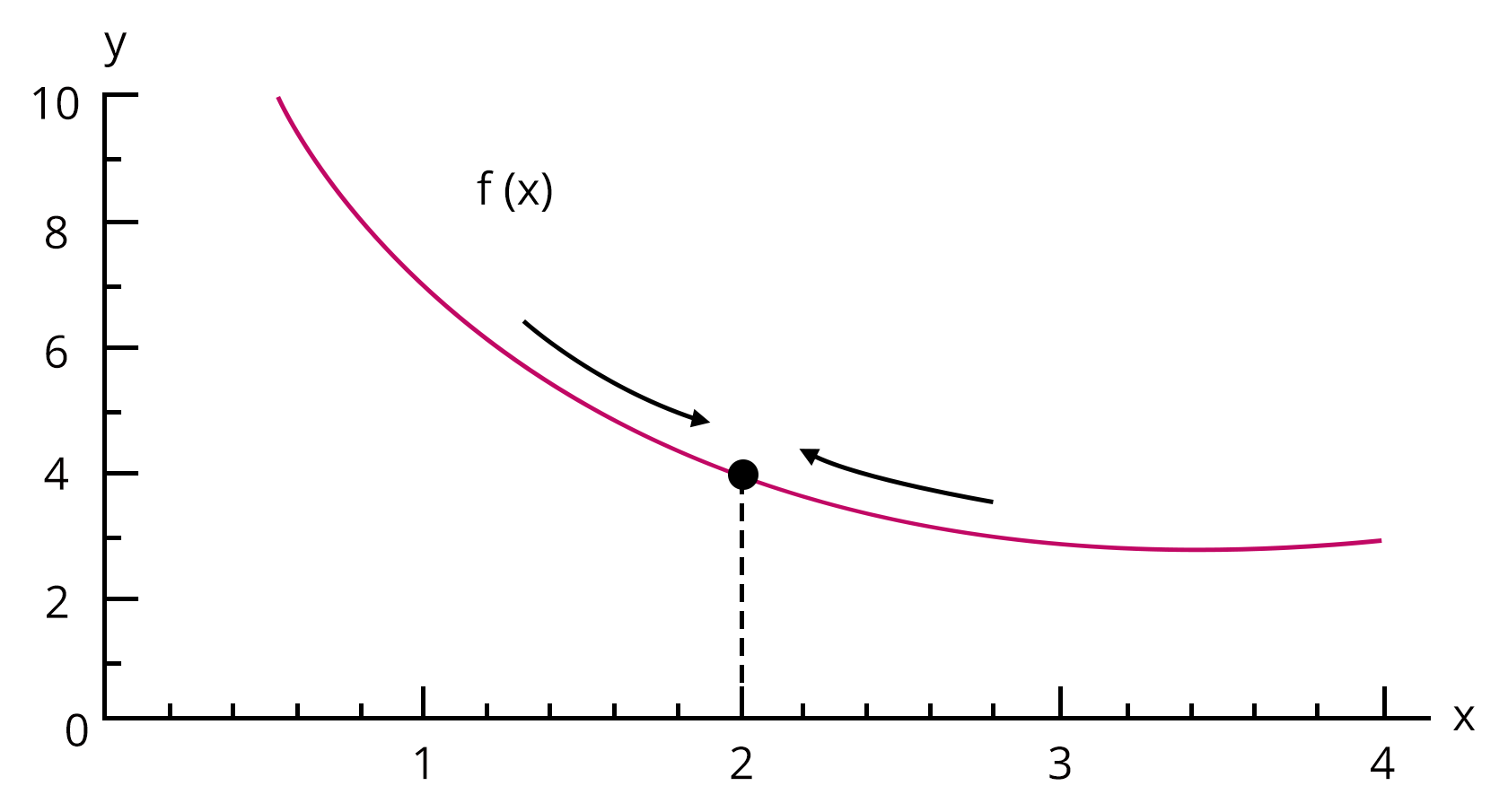### Existence of Limit, Left Hand Limit and Right Hand Limit - Types of Limits

Left Hand Limit - When a limit is described in terms of a number smaller than a, it is referred to as a left hand limit. It's written as $x \to a^{-}$, which is the same as $x=a-h$ with $h > 0$ and $h \to 0$.

Right Hand Limit - When a limit is described in terms of a number greater than a, it is referred to as a right hand limit. It is written as $x \to a^{+}$, which is the same as $x=a+h$ with $h > 0$ and $h \to 0$.

Existence of Limit - A function's limit at $x = a$ exists only if both its left and right hand limits exist, are equal, and have a finite value, i.e., $\underset{x \to a^{-}}{\lim} f(x) = \underset{x \to a^{+}}{\lim} f(x)$

### Limits and Functions

A function may reach one of two limits. One in which the variable approaches its limit by taking values that are greater than the limit, and the other in which the variable approaches its limit by taking values that are smaller. The limit is not stated in this situation, although the right and left-hand limitations do exist.

• When the $\underset{x \to a}{\lim} f(x) = A^{+}$ is used, the values of f near x to the right of ‘a’ are used. The right hand limit of f(x) at ‘a’ is stated to be this value.

• When the $\underset{x \to a}{\lim} f(x) = A^{-}$ is used, the values of f near x to the left of ‘a’ are used. The left hand limit of f(x) at ‘a’ is referred to as this value.

• The function’s limit exists if and only if the left-hand limit is equal to the right-hand limit.

### Important Limits Formula

1. $\underset{x \to 0}{\lim} \dfrac{\sin x}{x}=1$

2. $\underset{x \to 0}{\lim} \cos x=1$

3. $\underset{x \to 0}{\lim} \dfrac{\tan x}{x}=1$

4. $\underset{x \to 0}{\lim} \dfrac{1-\cos x}{x}=0$

5. $\underset{x \to 0}{\lim} \dfrac{\sin x^{\circ}}{x}=\dfrac{\pi}{180}$

6. $\underset{x \to a}{\lim} \dfrac{x^{n}-a^{n}}{x-a}=n a^{n-1}$

7. $\underset{x \to \infty}{\lim}\left(1+\dfrac{k}{x}\right)^{m x}=e^{m k}$

8. $\underset{x \to 0}{\lim}(1+x)^{\dfrac{1}{x}}=e$

9. $\underset{x \to 0}{\lim} \dfrac{\left(a^{x}-1\right)}{x}=\ln a$

10. $\underset{x \to 0}{\lim} \dfrac{e^{x}-1}{x}=1$

11.  $\underset{x \to 0}{\lim} \dfrac{\ln (1+x)}{x}=1$

12.  $\underset{x \to \infty}{\lim} x^{\dfrac{1}{x}}=1$

### L’Hospital Rule

If the supplied limit $\underset{x \to a}{\lim} \dfrac{f(x)}{g(x)}$ is of the form $\dfrac{0}{0}$ or $\dfrac{\infty}{\infty}$, that is, both f(x) and g(x) are 0 or both f(x) and g(x) are infinity, then the limit can be solved using the L'Hospital Rule that is,

$\underset{x \to a}{\lim} \dfrac{f(x)}{g(x)} = \underset{x \to a}{\lim} \dfrac{f^\prime(x)}{g^\prime(x)}$, where $f^\prime(x)$ and $g^\prime(x)$ is obtained by differentiating f(x) and g(x).

### What is Continuity and Differentiability?

The continuity of a function and the differentiability of a function are mutually exclusive. Before it can be shown for its differentiability at x = a, the function y = f(x) must first be proved for its continuity at x = a. Both continuity and differentiability can be demonstrated geometrically and algebraically.

### Continuity of a Function

The graph y = f(x) of a function is said to be continuous over a range if it is a single unbroken curve (the graph can be drawn easily without lifting the pencil at a point).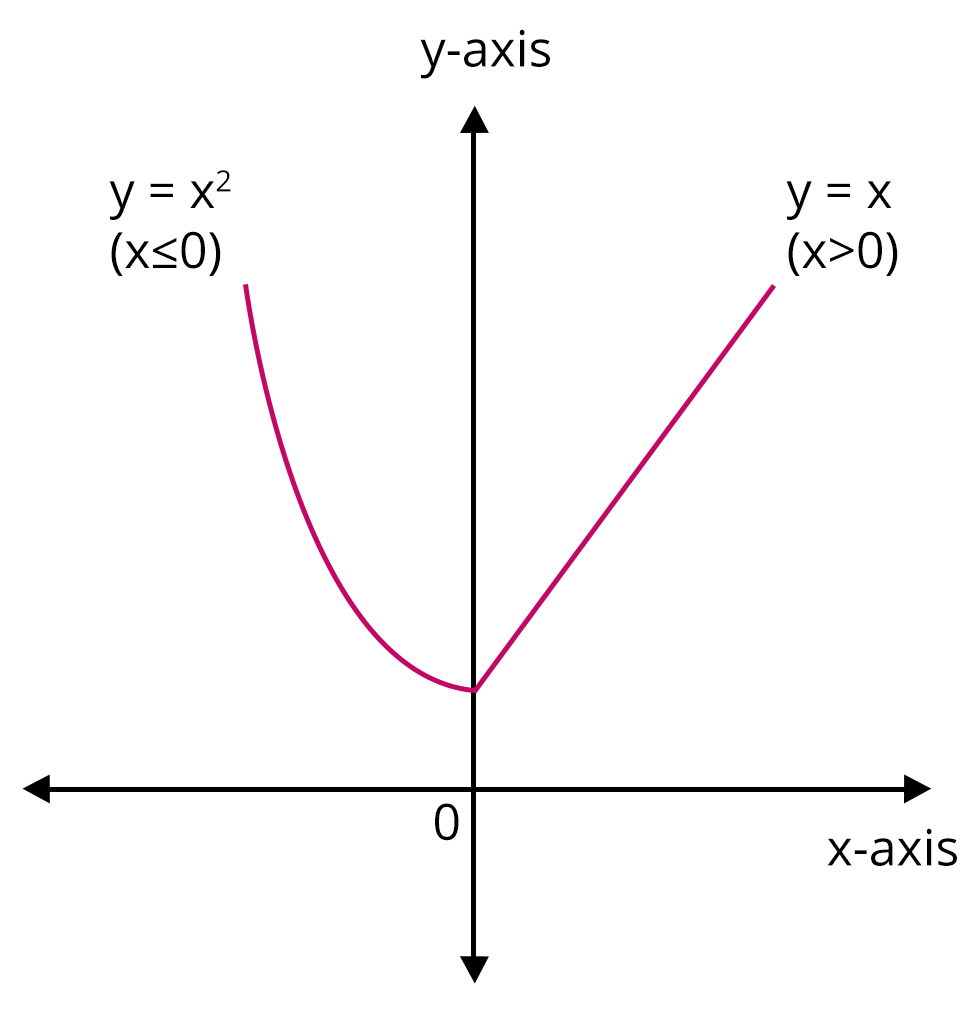Image: Graph of function y = f(x)

Technically we can say that, a continuous real valued function f(x) exists and is equal to $f(x_0)$ at a point $x=x_0$ in the domain if $\underset{x \to x_0}{\lim} f(x)$ exists and is equal to $f(x_0)$.

If a function f(x) is continuous at $x=x_0$, then $\underset{x \to x_0^{+}}{\lim} f(x) = \underset{x \to x_0^{-}}{\lim} f(x) = \underset{x \to x_0}{\lim} f(x)$

Discontinuous functions are those that are not continuous.

### Differentiability of A Function

The derivative of a real valued function f(x) with respect to x if the derivative $f^\prime(a)$ exists at every point in its domain, which is defined as

$\underset{h\to 0}{\lim} \dfrac{f(x+h)-f(x)}{h}$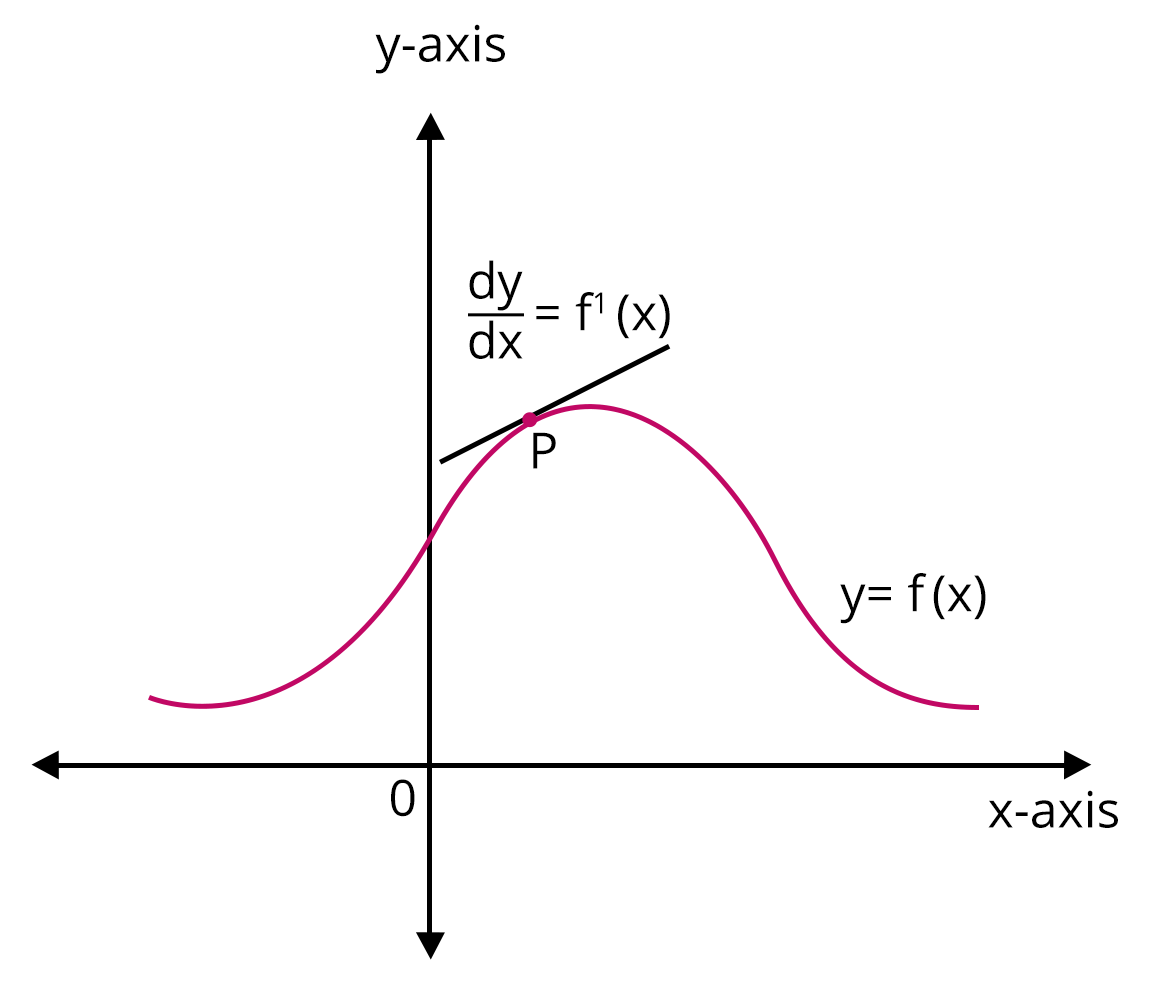Image: Derivative of a function f(x)

If the derivative of a function exists at all points in its domain, the function is said to be differentiable. To test the differentiability of a function at x=c, $\underset{h\to 0}{\lim} \dfrac{f(c+h)-f(c)}{h}$ must exist.

Thus we can say that every differentiable function is continuous

Note: If a function is differentiable at a given point, it is also continuous at that same point. But just because a function is continuous at a point doesn't mean it's also differentiable at that point.

### Important Differentiation of the Functions

Derivative of Composite Function

In Layman’s terms, to differentiate a composite function at any point in its domain, first differentiate the outer part (i.e., the function enclosing some other function) and then multiply it with the inner function’s derivative function. This will provide us with the desired differentiation.

Derivatives of Implicit Functions

When the dependent variable in a function is not explicitly isolated on either side of the equation, the function is referred to as an implicit function.

Derivatives of Inverse Trigonometric Functions

The derivatives of inverse trigonometric functions are known as inverse trigonometric derivatives. The inverses of the six basic trigonometric functions are called inverse trigonometric functions. Inverse trigonometric derivatives are defined only in the domain of inverse trigonometric functions, as follows:

 Inverse Trigonometric Function Domain arcsin x $\left[-1, 1\right]$ arccos x $\left[-1, 1\right]$ arctan x R arccosec x $(-\infty, -1] \cup [1, \infty)$ arcsec x $(-\infty, -1] \cup [1, \infty)$ arccot x R

Derivatives of Exponential Functions

The product of the exponential function ax and the natural log of a, $f^\prime(x) = ax~ln ~a$, is the derivative of the exponential function $f(x) = ax$, $a > 0$. The exponential function's derivative is written as $\dfrac{d(ax)}{dx} = (ax)^\prime = ax~ln~a$. The first principle of differentiation and the formulas of limits can be used to calculate the derivative of an exponential function. When $a > 1$ and when $a < 1$, the graph of the exponential function's derivative changes direction.

Formula for derivative of exponential function is given as,

$f(x) = ax, f^\prime(x) = ax~ln~a$ or $\dfrac{d(ax)}{dx} = ax~ln~a$

$f(x) = ex, f^\prime(x) = ex$ or $\dfrac{d(ex)}{dx} = ex$

Derivatives of Logarithmic Functions

The slope of the tangent to the curve representing the logarithmic function is determined by the logarithmic function's derivation. The following is the formula for the derivative of the common and natural logarithmic functions.

$\dfrac{1}{x}$ is the derivative of $ln x$. For example, $\dfrac{d}{dx} \dfrac{1}{x} = ln x$

$\dfrac{1}{x(x ~log~ a)}$ is the derivative of $log_a x$

Derivatives of Functions in Parametric Forms

There are times when we define a function using the third variable rather than explicitly or implicitly defining it. This is a parametric form, which is when a function y(x) is represented by a third variable called the parameter.

$\dfrac{d^2y}{dx^2} = \dfrac{d}{dx}\left(\dfrac{dy}{dx}\right) = \dfrac{\dfrac{d}{dt} \left(\dfrac{dy}{dx}\right)} {\dfrac{dx}{dt}}$

### Important Formulae of Continuity and Differentiability

1. $f(x)$ is continuous at $x=a$ if $\lim _{x \rightarrow a-} f(x)=\lim _{x \rightarrow a+} f(x)=f(a)$

2. $\dfrac{d}{d x}\left(x^{n}\right)=n x^{n-1}$ i.e $\dfrac{d}{d x}(\mathrm{x})=1$.

3. $\dfrac{d}{d x}(\sin x)=\cos x$.

4. $\dfrac{d}{d x}(\cos x)=-\sin x$.

5. $\cdot \dfrac{d}{d x}(\tan x)=\sec ^{2} x .$

6. $\dfrac{d}{d x}(\cot x)=-\operatorname{cosec}^{2} x$.

7. $\dfrac{d}{d x}(\sec x)=\sec x \tan x$.

8. $\dfrac{d}{d x}(\operatorname{cosec} x)=-\operatorname{cosec} x \cot x$.

9. $\dfrac{d}{d x}\left(\sin ^{-1} x\right)=\dfrac{1}{\sqrt{1-x^{2}}}$

10. $\dfrac{d}{d x}\left(\cos ^{-1} x\right)=-\dfrac{1}{\sqrt{1-x^{2}}}$

11. $\dfrac{d}{d x}\left(\tan ^{-1} x\right)=\dfrac{1}{1+x^{2}}$

12. $\dfrac{d}{d x}\left(\cot ^{-1} x\right)=-\dfrac{1}{1+x^{2}}$.

13. $\dfrac{d}{d x}\left(\sec ^{-1} x\right)=\dfrac{1}{x \sqrt{x^{2}-1}}$

14. $\dfrac{d}{d x}\left(\operatorname{cosec}^{-1} x\right)=-\dfrac{1}{x \sqrt{x^{2}-1}}$

### Theorems on Continuity and Differentiability

Theorem 1: If two functions f(x) and g(x) are continuous at a real valued function and continuous at a point x = c, we have:

At the point $c = c$, $f(x) + g(x)$ is continuous.

At $x = c$, $f(x) - g(x)$ is continuous.

Also at $x = c$, $g(x) \cdot g(x)$ is continuous.

At $x = c$, $\dfrac{f(x)}{g(x)}$ is continuous if $g(c) \neq 0$.

Theorem 2: The composite function fog(x) is defined at x = c for two real-valued functions f(x) and g(x). If the function f(x) is continuous at g(c) and g(x) is continuous at x = c, then fog(x) is continuous at x = c.

Theorem 3: If a function f(x) is differentiable at x = c, it is also continuous at that point. Every differentiable function is continuous, in other words.

Theorem 4: Chain Rule - $f = vou$ for a real-valued function f(x) is a composite of two functions u and v. Also, if $t = u(x)$ and both $\dfrac{dt}{dx}$ and $\dfrac{dv}{dt}$ exist, then $\dfrac{df}{dx} = \dfrac{dv}{dt}\cdot dt \cdot dx$ is obtained.

Theorem 5: The derivative of $e^x$ with respect to $x$ is $e^x$. And the derivative of $log x$ with respect to $x$ is $\dfrac{1}{x}$.

Theorem 6: Rolle's Theorem - If a function f(x) is continuous across the interval $\left[a, b\right]$ and differentiable across the interval (a, b), such that f(a) = f(b), and a, b are some real numbers. Then there exists a point c in the interval $\left[a, b\right]$ such that $f^\prime(c) = 0$.

Theorem 7: Mean Value Theorem - If a function f(x) is continuous across the interval $\left[a, b\right]$ and differentiable across the interval (a, b), then there exists a point c in the interval $\left[a, b\right]$ such that $f^\prime(c) = \dfrac{f(b) - f(a)}{b - a}$.

### Applications of Derivatives:

1. Rate of Change Formula

The rate of change function represents the rate at which one quantity changes in relation to another. Simply put, the rate of change is the difference between the amount of change in one item and the corresponding amount of change in another.

i.e., $\dfrac{\Delta y}{\Delta x} = \dfrac{y_2 - y_1}{x_2 - x_1}$

For a linear function

$\dfrac{\Delta y}{\Delta x} = \dfrac{f(b) - f(a)}{b - a}$

Note:

The rate of change of quantity, $\dfrac{dy}{dx}$, is represented by a positive sign when it increases.

The rate of change of quantity, $\dfrac{dy}{dx}$, is represented by a negative sign when it decreases.

2. Increasing and Decreasing Functions

If the value of y increases as the value of x increases, then the function $y = F(x)$ is known as an increasing function, and if the value of y decreases as the value of x increases, then the function $y = F(x)$ is known as a decreasing function.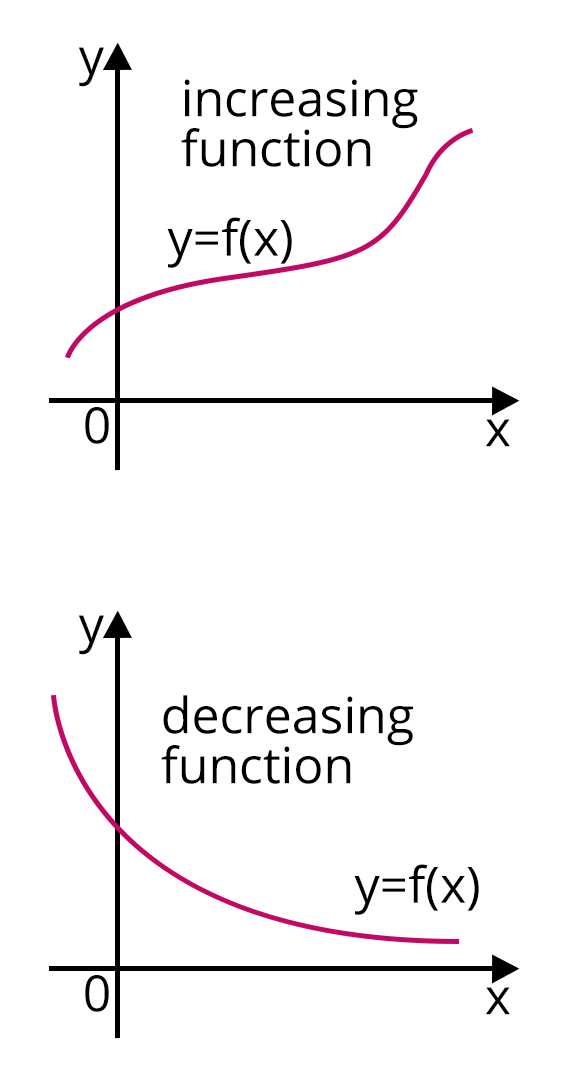Image: Increasing and Decreasing Function

3. Maxima and Minima of Functions

The extrema of a function are its maximum and minimum values. The maximum and minimum values of a function within a given set of ranges are known as maxima and minima. The maximum value of the function under the entire range is known as the absolute maxima, and the minimum value is known as the absolute minima.

In the diagram below, we get maximum values of the function at x = a and x = 0, and minimum values of the function at x = b and x = c. The maxima are all the peaks, and the minima are all the valleys.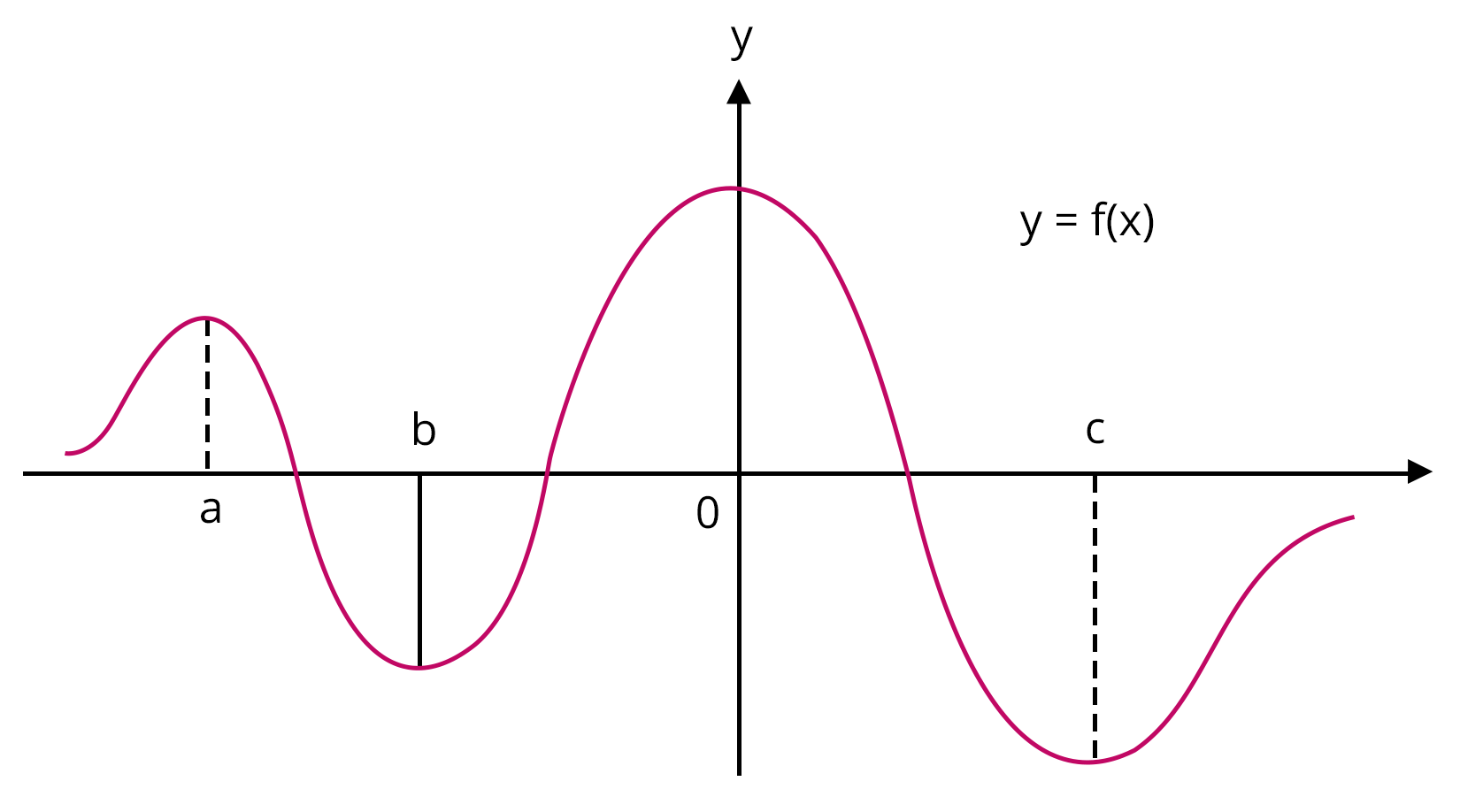Image: Maxima and Minima

4. Tangents and Normals

A tangent to a curve is a line that touches it at one point and has the same slope as the curve.

A line perpendicular to a tangent to the curve is called a normal to the curve.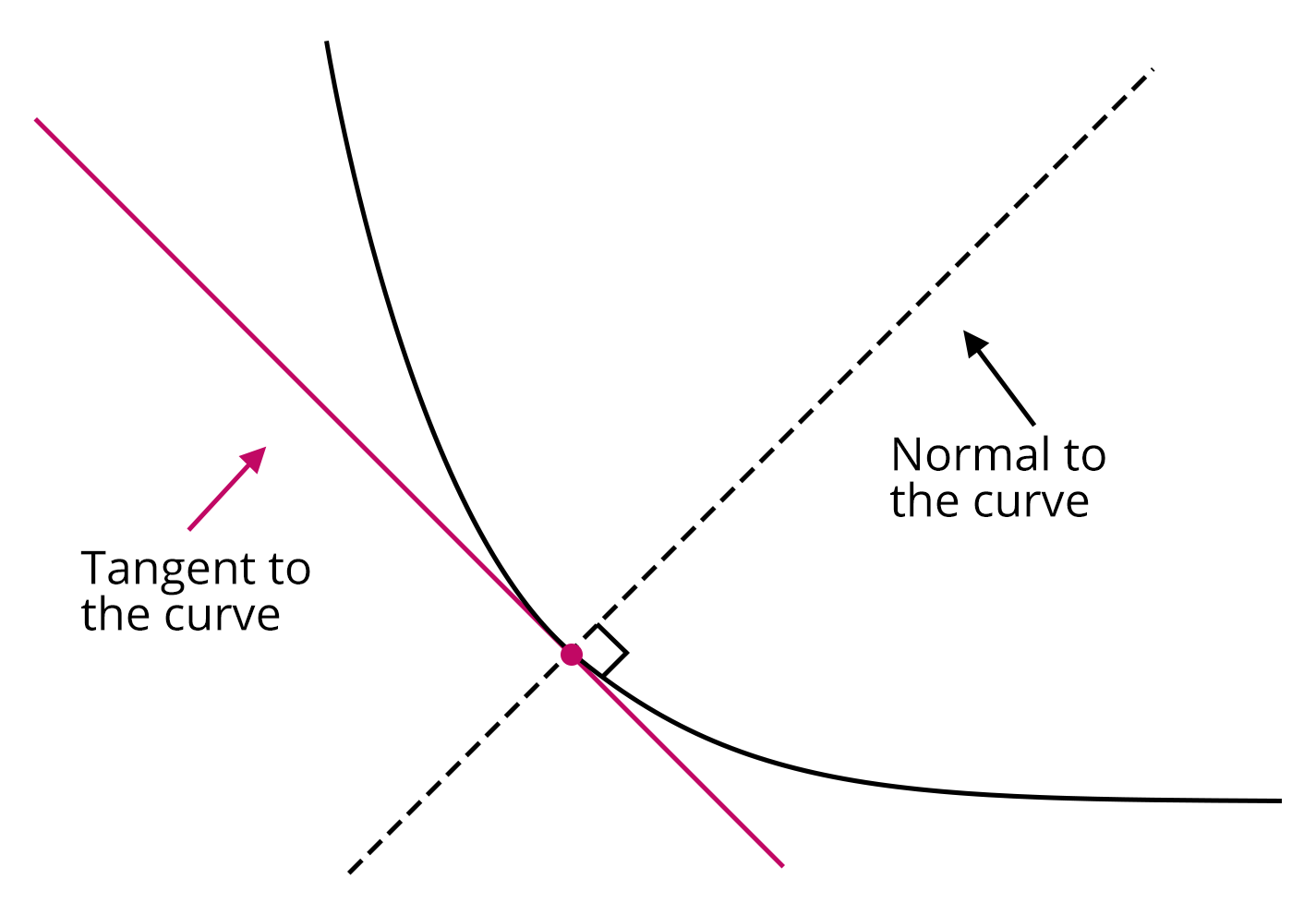Image: Tangent and Normal

### Examples on Limit and Continuity

Example on Limit:

Evaluate $\underset{x\to 0}{\lim} \dfrac{x\cos{(x)}-\sin{(x)}}{x^2\sin{(x)}}$

Solution: Here the given limit is of the form $\dfrac{0}{0}$

Using L’Hospital Rule and differentiating, we get

$\Rightarrow \underset{x \to 0}{\lim} \dfrac{\cos{(x)}-x\sin{(x)}-\cos{(x)}}{x^2\cos{(x)} + 2x\sin{(x)}}$

$\Rightarrow \underset{x \to 0}{\lim} \dfrac{-\sin{(x)}}{x\cos{(x)} + 2\sin{(x)}}$

$\Rightarrow \underset{x \to 0}{\lim} \dfrac{\dfrac{-\sin{(x)}}{x}}{\cos{(x)} + \dfrac{2\sin{(x)}}{x}}$

$\Rightarrow \dfrac{-1}{1 + 2(1)}$

$\Rightarrow \dfrac{-1}{3}$

Example on Continuity:

Find the continuity of the function f(x) = 3x + 4 at the point x = 5.

Solution: The given function is f(x) = 3x + 4, and its value at the point x = 5 is f(5) = 19.

Let us find the limit of the function at the point x = 5.

$\underset{x \to 5}{\lim}f(x)$

$\Rightarrow \underset{x \to 5}{\lim} (3x + 4)$

$\Rightarrow 3(5) + 4$

$\Rightarrow 15 + 4 = 19 = f(5)$

Therefore, the function f(x) is continuous at the point x = 5.

### Solved problems of Previous Year’s Question

1. If $f(1)=1, f^{\prime}(1)=2$ then $\underset{x \rightarrow 1}{\lim} \dfrac{\sqrt{f(x)}-1}{\sqrt{x}-1}$ is

Ans: Given function is $\underset{x \rightarrow 1}{\lim} \dfrac{\sqrt{f(x)}-1}{\sqrt{x}-1}$ and the limit is of the form (0/0)

Using L’Hospital's rule we get,

$\Rightarrow \underset{x \rightarrow 1}{\lim} \dfrac{\dfrac{1}{2 \sqrt{f(x)}} f^{\prime}(x)}{\dfrac{1}{2 \sqrt{x}}}$

$\Rightarrow \dfrac{f^{\prime}(1)}{\sqrt{f(1)}}$

As it is given that $f(1)=1, f^{\prime}(1)=2$

Hence $\dfrac{f^{\prime}(1)}{\sqrt{f(1)}}=2$

2. $f(x)$ and $g(x)$ are two differentiable functions on $\left[0,2\right]$ such that $f^{\prime \prime}(x)-g^{\prime \prime}(x)=0$, $f^{\prime \prime}(1)=2 g^{\prime}(1)=4 f(2)=3 g(2)=9$ then $f(x)-g(x)$ at $x=3 / 2$ is

Ans: Given $f^{\prime \prime}(x)-g^{\prime \prime}(x)=0$

On Integrating the above equation we get,

$f^{\prime}(x)-g^{\prime}(x)=c$;  \Rightarrow f^{\prime}(1)-g^{\prime}(1)=c\Rightarrow 4-2 = c  \Rightarrow c=2\therefore f^{\prime}(x)-g^{\prime}(x)=2$Again Integrating the above equation to reduce to f(x) and g(x) we get,$f(x)-g(x)=2 x+c_{1}\Rightarrow f(2)-g(2)=4+c_{1}\Rightarrow 9-3=4+c_{1} ; \Rightarrow c_{1}=2\therefore f(x)-g(x)=2 x+2$at$x= \dfrac{3}{2}, f(x)-g(x)=3+2=5$. 3. Solve the limit$\underset{x \rightarrow \alpha}{\lim} \dfrac{1-\cos a(x-\alpha)(x-\beta)}{(x-\alpha)^{2}}$Ans: Given,$\underset{x \rightarrow \alpha}{\lim} \dfrac{1-\cos a(x-\alpha)(x-\beta)}{(x-\alpha)^{2}}$We know that$1-cos\theta = 2sin^2\theta$Thus the given equation will become,$\Rightarrow \lim _{x \rightarrow \alpha} \dfrac{2 \sin ^{2}\left(a \dfrac{(x-\alpha)(x-\beta)}{2}\right)}{(x-\alpha)^{2}}\Rightarrow \lim _{x \rightarrow \alpha} \dfrac{2}{(x-\alpha)^{2}} \times \dfrac{\sin ^{2}\left(a\dfrac{(x-\alpha)(x-\beta)}{2}\right)} {\dfrac{a^{2}(x-\alpha)^{2}(x-\beta)^{2}}{4}}\times \dfrac{a^{2}(x-\alpha)^{2}(x-\beta)^{2}}{4}\Rightarrow \dfrac{a^{2}(\alpha-\beta)^{2}}{2}$### Practice Questions 1. Let$f(x)=4$and$f^{\prime}(x)=4$. Then$\underset{x \rightarrow 2}{\lim} \dfrac{x f(2)-2 f(x)}{x-2}$is given by Ans: - 4 Hint: Apply L’Hospital rule 2. If$f(x+y)=f(x) . f(y) \forall x, y$and$f(5)=2, f^{\prime}(0)=$3 then$f^{\prime}(5)$is Ans: 6 Hint: Differentiate with respect to x, treating y as constant ### Conclusion Some of the important concepts covered in this chapter include limits, functions, differentiability, continuity, and L’Hospital Rule. It is one of the important chapters in class 12 and it can be continued in higher classes also. Many questions will be asked from this chapter in and JEE Advanced both. So the students preparing for JEE must practice the questions from this chapter. ## Important Related Links for JEE Main 2022-23 See More## JEE Main Important Dates View all JEE Main Exam Dates JEE Main 2023 January and April Session exam dates and revised schedule have been announced by the NTA. JEE Main 2023 January and April Session will now be conducted on 24-Jan-2023 to 31-Jan-2023 and 6-Apr-2023 to 12-Apr-2023, and the exam registration closes on 12-Jan-2023 and Apr-2023. You can check the complete schedule on our site. Furthermore, you can check JEE Main 2023 dates for application, admit card, exam, answer key, result, counselling, etc along with other relevant information. See More View all JEE Main Exam Dates## JEE Main Information Last updated date: 15th Mar 2023 Total views: 87.9k Views today: 10 Application Form Eligibility Criteria Reservation Policy Admit Card Exam Centres NTA has announced the JEE Main 2023 January session application form release date on the official website https://jeemain.nta.nic.in/. JEE Main 2023 January and April session Application Form is available on the official website for online registration. Besides JEE Main 2023 January and April session application form release date, learn about the application process, steps to fill the form, how to submit, exam date sheet etc online. Check our website for more details. April Session's details will be updated soon by NTA.## JEE Main Syllabus View JEE Main Syllabus in Detail Last updated date: 15th Mar 2023 Total views: 87.9k Views today: 10 It is crucial for the the engineering aspirants to know and download the JEE Main 2023 syllabus PDF for Maths, Physics and Chemistry. Check JEE Main 2023 syllabus here along with the best books and strategies to prepare for the entrance exam. Download the JEE Main 2023 syllabus consolidated as per the latest NTA guidelines from Vedantu for free. See More Download full syllabus Download full syllabus View JEE Main Syllabus in Detail## JEE Main 2023 Study Material View all study material for JEE Main JEE Main 2023 Study Materials: Strengthen your fundamentals with exhaustive JEE Main Study Materials. It covers the entire JEE Main syllabus, DPP, PYP with ample objective and subjective solved problems. Free download of JEE Main study material for Physics, Chemistry and Maths are available on our website so that students can gear up their preparation for JEE Main exam 2023 with Vedantu right on time. See More All Mathematics Physics Chemistry See All## JEE Main Question Papers see all Download JEE Main Question Papers & ​Answer Keys of 2022, 2021, 2020, 2019, 2018 and 2017 PDFs. JEE Main Question Paper are provided language-wise along with their answer keys. We also offer JEE Main Sample Question Papers with Answer Keys for Physics, Chemistry and Maths solved by our expert teachers on Vedantu. Downloading the JEE Main Sample Question Papers with solutions will help the engineering aspirants to score high marks in the JEE Main examinations. See More## JEE Main 2023 Book Solutions and PDF Download View all JEE Main Important Books Last updated date: 15th Mar 2023 Total views: 87.9k Views today: 10 In order to prepare for JEE Main 2023, candidates should know the list of important books i.e. RD Sharma Solutions, NCERT Solutions, RS Aggarwal Solutions, HC Verma books and RS Aggarwal Solutions. They will find the high quality readymade solutions of these books on Vedantu. These books will help them in order to prepare well for the JEE Main 2023 exam so that they can grab the top rank in the all India entrance exam. See More Maths NCERT Book for Class 12 Maths Physics NCERT Book for Class 12 Physics Chemistry NCERT Book for Class 12 Chemistry Physics H. C. Verma Solutions Maths R. D. Sharma Solutions Maths R.S. Aggarwal Solutions See All## JEE Main Mock Tests View all mock tests JEE Main 2023 free online mock test series for exam preparation are available on the Vedantu website for free download. Practising these mock test papers of Physics, Chemistry and Maths prepared by expert teachers at Vedantu will help you to boost your confidence to face the JEE Main 2023 examination without any worries. The JEE Main test series for Physics, Chemistry and Maths that is based on the latest syllabus of JEE Main and also the Previous Year Question Papers. See More## JEE Main 2023 Cut-Off JEE Main Cut Off Last updated date: 15th Mar 2023 Total views: 87.9k Views today: 10 NTA is responsible for the release of the JEE Main 2023 January and April Session cut off score. The qualifying percentile score might remain the same for different categories. According to the latest trends, the expected cut off mark for JEE Main 2023 January and April Session is 50% for general category candidates, 45% for physically challenged candidates, and 40% for candidates from reserved categories. For the general category, JEE Main qualifying marks for 2021 ranged from 87.8992241 for general-category, while for OBC/SC/ST categories, they ranged from 68.0234447 for OBC, 46.8825338 for SC and 34.6728999 for ST category. See More## JEE Main 2023 Results Last updated date: 15th Mar 2023 Total views: 87.9k Views today: 10 NTA will release the JEE Main 2023 January and April sessions exam dates on the official website, i.e. {official-website}. Candidates can directly check the date sheet on the official website or https://jeemain.nta.nic.in/. JEE Main 2023 January and April sessions is expected to be held in February and May. Visit our website to keep updates of the respective important events of the national entrance exam. See More Rank List Counselling Cutoff JEE Main 2023 state rank lists will be released by the state counselling committees for admissions to the 85% state quota and to all seats in IIT colleges. JEE Main 2023 state rank lists are based on the marks obtained in entrance exams. Candidates can check the JEE Main 2023 state rank list on the official website or on our site.## JEE Top Colleges View all JEE Main 2023 Top Colleges Last updated date: 15th Mar 2023 Total views: 87.9k Views today: 10 Want to know which Engineering colleges in India accept the JEE Main 2023 scores for admission to Engineering? Find the list of Engineering colleges accepting JEE Main scores in India, compiled by Vedantu. There are 1622 Colleges that are accepting JEE Main. Also find more details on Fees, Ranking, Admission, and Placement. See More## FAQs on JEE - Limit, Continuity and Differentiability FAQ 1. Mention the most important differentiation of the functions f(x) based on the type of functions. Some of the most important differentiation of the functions f(x) based on the type of functions are given below: • Derivative of Composite Function • Derivatives of Implicit Functions • Derivatives of Inverse Trigonometric Functions • Derivatives of Exponential Functions • Derivatives of Logarithmic Functions • Derivatives of Functions in Parametric Forms 2. What Are the Applications of Calculus Limits in Real Life? Limits are also utilized in practice to approximate the calculation of derivatives. Engineers will estimate a function using small changes in the function and then try to determine the derivative of the function using smaller spacing in the function following sample intervals in order to do computations. 3. Mention two of the most important theorems on continuity and differentiability Rolle's Theorem - If a function f(x) is continuous across the interval$\left[a, b\right]$and differentiable across the interval (a, b), such that f(a) = f(b), and a, b are some real numbers. Then there exists a point c in the interval$\left[a, b\right]$such that$f^\prime(c) = 0$. Mean Value Theorem - If a function f(x) is continuous across the interval$\left[a, b\right]$and differentiable across the interval (a, b), then there exists a point c in the interval$\left[a, b\right]$such that$f^\prime(c) = \dfrac{f(b) - f(a)}{b - a}\$.## JEE Main Upcoming Dates

Vedantu offers free live Master Classes for CBSE Class 6 to 12, ICSE, JEE Main, JEE 2023, & more by India’s best teachers. Learn all the important concepts concisely along with amazing tricks to score high marks in your class and other competitive exams.
See MoreJEE Main Exam April Session Starts
06 May 202346 days remainingJEE Main Exam April Session Ends
12 May 202353 days remaining## Notice board

JEE News
JEE BlogsTrending pages